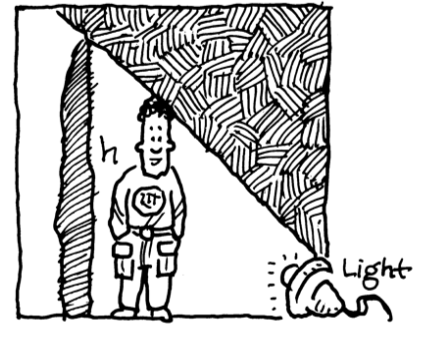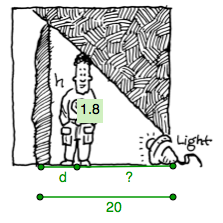### Home > PC > Chapter 10 > Lesson 10.1.3 > Problem10-43

10-43.

Greg’s shadow is cast on a wall by a light on the ground which is $20$ meters from the wall. Greg is $180$ centimeters tall. Express the height (in meters) of his shadow, $h$, as a function of his distance from the wall, $d$.Draw and label a diagram.

See diagram at right.
What expression would you use for the '?' ?Write a proportion using the similar triangles in the diagram.

$\frac{1.8}{20-\textit{d}}=\frac{\textit{h}}{20}$

Now rewrite the equation in $h =$ form.